# NCERT solutions for Mathematics Exemplar Class 6 chapter 8 - Ratio and Proportion [Latest edition]

#### Chapters## Chapter 8: Ratio and Proportion

Exercise
Exercise [Pages 120 - 130]

### NCERT solutions for Mathematics Exemplar Class 6 Chapter 8 Ratio and Proportion Exercise [Pages 120 - 130]

#### Choose the correct alternative:

Exercise | Q 1 | Page 120

The ratio of 8 books to 20 books is ______.

• 2:5

• 5:2

• 4:5

• 5:4

Exercise | Q 2 | Page 120

The ratio of the number of sides of a square to the number of edges of a cube is ______.

• 1:2

• 3:2

• 4:1

• 1:3

Exercise | Q 3 | Page 120

A picture is 60 cm wide and 1.8 m long. The ratio of its width to its perimeter in lowest form is ______.

• 1:2

• 1:3

• 1:4

• 1:8

Exercise | Q 4 | Page 120

Neelam’s annual income is Rs. 288000. Her annual savings amount to Rs. 36000. The ratio of her savings to her expenditure is ______.

• 1:8

• 1:7

• 1:6

• 1:5

Exercise | Q 5 | Page 120

Mathematics textbook for Class VI has 320 pages. The chapter ‘symmetry’ runs from page 261 to page 272. The ratio of the number of pages of this chapter to the total number of pages of the book is ______.

• 11:320

• 3:40

• 3:80

• 272:320

Exercise | Q 6 | Page 120

In a box, the ratio of red marbles to blue marbles is 7:4. Which of the following could be the total number of marbles in the box?

• 18

• 19

• 21

• 22

Exercise | Q 7 | Page 121

On a shelf, books with green cover and that with brown cover are in the ratio 2:3. If there are 18 books with green cover, then the number of books with brown cover is ______.

• 12

• 24

• 27

• 36

Exercise | Q 8 | Page 121

The greatest ratio among the ratios 2:3, 5:8, 75:121 and 40:25 is ______.

• 2:3

• 5:8

• 75:121

• 40:25

Exercise | Q 9 | Page 121

There are ‘b’ boys and ‘g’ girls in a class. The ratio of the number of boys to the total number of students in the class is ______.

• b/(b + g)

• g/(b + g)

• b/g

• (b + g)/b

Exercise | Q 10 | Page 121

If a bus travels 160 km in 4 hours and a train travels 320 km in 5 hours at uniform speeds, then the ratio of the distances travelled by them in one hour is ______.

• 1:2

• 4:5

• 5:8

• 8:5

Exercise | Q 11 | Page 121

Find the missing number in the box  in the proportions:

3/8 = square/20

Exercise | Q 12 | Page 121

Find the missing number in the box  in the proportions:

square/18 = 2/9

Exercise | Q 13 | Page 121

Find the missing number in the box  in the proportions:

8/square = 3.2/4

Exercise | Q 14 | Page 121

Find the missing number in the box  in the proportions:

square/45 = 16/40 = 24/square

Exercise | Q 15 | Page 121

Find the missing number in the box  in the proportions:

16/36 = square/63 = 36/square = square/117

#### State whether the following statement is True or False:

Exercise | Q 16 | Page 121

3/8 = 15/40

• True

• False

Exercise | Q 17 | Page 121

4:7 = 20:35

• True

• False

Exercise | Q 18 | Page 121

0.2:5 = 2:0.5

• True

• False

Exercise | Q 19 | Page 121

3:33 = 33:333

• True

• False

Exercise | Q 20 | Page 121

15 m : 40 m = 35 m : 65 m

• True

• False

Exercise | Q 21 | Page 121

27 cm2 : 57 cm2 = 18 cm : 38 cm

• True

• False

Exercise | Q 22 | Page 121

5 kg : 7.5 kg = Rs 7.50 : Rs 5

• True

• False

Exercise | Q 23 | Page 121

20 g : 100 g = 1 metre : 500 cm

• True

• False

Exercise | Q 24 | Page 121

12 hours : 30 hours = 8 km : 20 km

• True

• False

Exercise | Q 25 | Page 121

The ratio of 10 kg to 100 kg is 1:10

• True

• False

Exercise | Q 26 | Page 121

The ratio of 150 cm to 1metre is 1:1.5.

• True

• False

Exercise | Q 27 | Page 121

25 kg : 20 g = 50 kg : 40 g

• True

• False

Exercise | Q 28 | Page 121

The ratio of 1 hour to one day is 1:1.

• True

• False

Exercise | Q 29 | Page 121

The ratio 4:16 is in its lowest form.

• True

• False

Exercise | Q 30 | Page 121

The ratio 5:4 is different from the ratio 4:5.

• True

• False

Exercise | Q 31 | Page 121

A ratio will always be more than 1.

• True

• False

Exercise | Q 32 | Page 121

A ratio can be equal to 1.

• True

• False

Exercise | Q 33 | Page 121

If b : a = c : d, then a, b, c, d are in proportion.

• True

• False

Exercise | Q 34 | Page 121

The two terms of a ratio can be in two different units.

• True

• False

#### Fill in the blank:

Exercise | Q 35 | Page 122

A ratio is a form of comparison by ______.

Exercise | Q 36 | Page 122

20 m : 70 m = Rs 8 : Rs ______.

Exercise | Q 37 | Page 122

There is a number in the box square such that square, 24, 9, 12 are in proportion. The number in the box is ______.

Exercise | Q 38 | Page 122

If two ratios are equal, then they are in ______.

#### Use following figure (in which each square is unit of length)

Exercise | Q 39 | Page 123

The ratio of the perimeter of the boundary of the shaded portion to the perimeter of the whole figure is ______.

Exercise | Q 40 | Page 123

The ratio of the area of the shaded portion to that of the whole figure is ______.

Exercise | Q 41 | Page 123

Sleeping time of a python in a 24 hour clock is represented by the shaded portion in figure.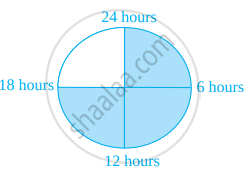The ratio of sleeping time to awaking time is ______.

Exercise | Q 42 | Page 123

A ratio expressed in lowest form has no common factor other than ______ in its terms.

Exercise | Q 43 | Page 123

To find the ratio of two quantities, they must be expressed in _____ units.

Exercise | Q 44 | Page 123

Ratio of 5 paise to 25 paise is the same as the ratio of 20 paise to ______.

Exercise | Q 45 | Page 123

Saturn and Jupiter take 9 hours 56 minutes and 10 hours 40 minutes, respectively for one spin on their axes. The ratio of the time taken by Saturn and Jupiter in lowest form is ______.

Exercise | Q 46 | Page 123

10 g of caustic soda dissolved in 100 mL of water makes a solution of caustic soda. Amount of caustic soda needed for 1 litre of water to make the same type of solution is ______.

Exercise | Q 47 | Page 123

The marked price of a table is Rs. 625 and its sale price is Rs. 500. What is the ratio of the sale price to the marked price?

Exercise | Q 48.(i) | Page 124

Which pair of ratios are equal? And why?

2/3, 4/6

Exercise | Q 48.(ii) | Page 124

Which pair of ratios are equal? And why?

8/4, 2/1

Exercise | Q 48.(iii) | Page 124

Which pair of ratios are equal? And why?

4/5, 12/50

Exercise | Q 49 | Page 124

Which ratio is larger 10 : 21 or 21 : 93?

Exercise | Q 50 | Page 124

Reshma prepared 18 kg of Burfi by mixing Khoya with sugar in the ratio of 7:2. How much Khoya did she use?

Exercise | Q 51 | Page 124

A line segment 56 cm long is to be divided into two parts in the ratio of 2:5. Find the length of each part.

Exercise | Q 52 | Page 124

The number of milk teeth in human beings is 20 and the number of permanent teeth is 32. Find the ratio of the number of milk teeth to the number of permanent teeth.

Exercise | Q 53 | Page 124

Sex ratio is defined as the number of females per 1000 males in the population. Find the sex ratio if there are 3732 females per 4000 males in a town.

Exercise | Q 54.(a) | Page 124

In a year, Ravi earns Rs 360000 and paid Rs 24000 as income tax. Find the ratio of his income to income tax.

Exercise | Q 54.(b) | Page 124

In a year, Ravi earns Rs 360000 and paid Rs 24000 as income tax. Find the ratio of his income tax to income after paying income tax.

Exercise | Q 55.(a) | Page 124

Ramesh earns Rs. 28000 per month. His wife Rama earns Rs. 36000 per month. Find the ratio of ramesh’s earnings to their total earnings

Exercise | Q 55.(b) | Page 124

Ramesh earns Rs. 28000 per month. His wife Rama earns Rs. 36000 per month. Find the ratio of rama’s earnings to their total earnings.

Exercise | Q 56.(a) | Page 124

Of the 288 persons working in a company, 112 are men and the remaining are women. Find the ratio of the number of men to that of women.

Exercise | Q 56.(b) | Page 124

Of the 288 persons working in a company, 112 are men and the remaining are women. Find the ratio of the number of men to the total number of persons.

Exercise | Q 56.(c) | Page 124

Of the 288 persons working in a company, 112 are men and the remaining are women. Find the ratio of the number of women to the total number of persons.

Exercise | Q 57 | Page 124

A rectangular sheet of paper is of length 1.2 m and width 21 cm. Find the ratio of width of the paper to its length.

Exercise | Q 58 | Page 125

A scooter travels 120 km in 3 hours and a train travels 120 km in 2 hours. Find the ratio of their speeds.

Exercise | Q 59 | Page 125

An office opens at 9 a.m. and closes at 5.30 p.m. with a lunch break of 30 minutes. What is the ratio of lunch break to the total period in the office?

Exercise | Q 60 | Page 125

The shadow of a 3 m long stick is 4m long. At the same time of the day, if the shadow of a flagstaff is 24 m long, how tall is the flagstaff?

Exercise | Q 61 | Page 125

A recipe calls for 1 cup of milk for every 2 1/2 cups of flour to make a cake that would feed 6 persons. How many cups of both flour and milk will be needed to make a similar cake for 8 people?

Exercise | Q 62 | Page 125

In a school, the ratio of the number of large classrooms to small classrooms is 3:4. If the number of small rooms is 20, then find the number of large rooms.

Exercise | Q 63.(a) | Page 125

Samira sells newspapers at Janpath crossing daily. On a particular day, she had 312 newspapers out of which 216 are in English and remaining in Hindi. Find the ratio of the number of English newspapers to the number of Hindi newspapers.

Exercise | Q 63.(b) | Page 125

Samira sells newspapers at Janpath crossing daily. On a particular day, she had 312 newspapers out of which 216 are in English and remaining in Hindi. Find the ratio of the number of Hindi newspapers to the total number of newspapers.

Exercise | Q 64.(a) | Page 125

The students of a school belong to different religious backgrounds. The number of Hindu students is 288, the number of Muslim students is 252, the number of Sikh students is 144 and the number of Christian students is 72. Find the ratio of the number of Hindu students to the number of Christian students.

Exercise | Q 64.(b) | Page 125

The students of a school belong to different religious backgrounds. The number of Hindu students is 288, the number of Muslim students is 252, the number of Sikh students is 144 and the number of Christian students is 72. Find the ratio of the number of Muslim students to the total number of students.

Exercise | Q 65 | Page 126

When Chinmay visted chowpati at Mumbai on a holiday, he observed that the ratio of North Indian food stalls to South Indian food stalls is 5:4. If the total number of food stalls is 117, find the number of each type of food stalls.

Exercise | Q 66 | Page 126

At the parking stand of Ramleela ground, Kartik counted that there are 115 cycles, 75 scooters and 45 bikes. Find the ratio of the number of cycles to the total number of vehicles.

Exercise | Q 67 | Page 126

A train takes 2 hours to travel from Ajmer to Jaipur, which are 130 km apart. How much time will it take to travel from Delhi to Bhopal which are 780 km apart if the train is travelling at the uniform speed?

Exercise | Q 68 | Page 126

The length and breadth of a school ground are 150 m and 90 m respectively, while the length and breadth of a mela ground are 210 m and 126 m, respectively. Are these measurements in proportion?

Exercise | Q 69 | Page 127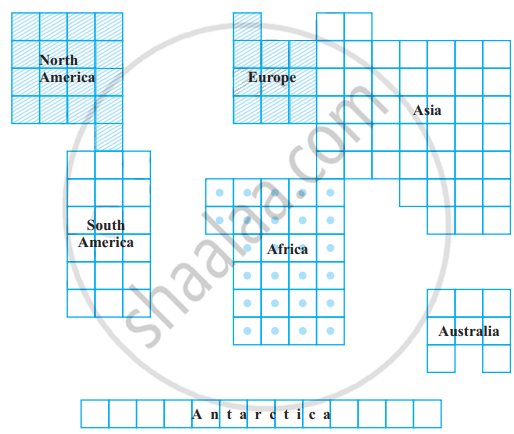In the given figure, the comparative areas of the continents are given: What is the ratio of the areas of
(a) Africa to Europe
(b) Australia to Asia
(c) Antarctica to Combined area of North America and South America.

Exercise | Q 70 | Page 127

A tea merchant blends two varieties of tea costing her Rs. 234 and Rs. 130 per kg in the ratio of their costs. If the weight of the mixture is 84 kg, then find the weight of each variety of tea.

Exercise | Q 71 | Page 127

An alloy contains only zinc and copper and they are in the ratio of 7:9. If the weight of the alloy is 8 kg, then find the weight of copper in the alloy.

Exercise | Q 72.(i) | Page 127

In the following figure, division represents 1 cm: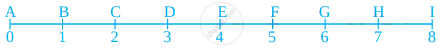Express numerically the ratios of the following distances:
AC : AF

Exercise | Q 72.(ii) | Page 127

In the following figure, division represents 1 cm:Express numerically the ratios of the following distances:

Exercise | Q 72.(iii) | Page 127

In the following figure, division represents 1 cm:Express numerically the ratios of the following distances:
BF : AI

Exercise | Q 72.(iv) | Page 127

In the following figure, division represents 1 cm:Express numerically the ratios of the following distances:
CE : DI

Exercise | Q 73 | Page 127

Find two numbers whose sum is 100 and whose ratio is 9:16.

Exercise | Q 74.(i) | Page 127

In figure, find the ratio of the area of the shaded portion to that of the whole figure: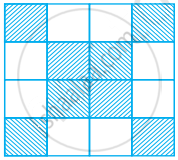Exercise | Q 74.(b) | Page 127

In figure, find the ratio of the area of the shaded portion to that of the whole figure: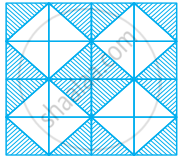Exercise | Q 75 | Page 127

A typist has to type a manuscript of 40 pages. She has typed 30 pages of the manuscript. What is the ratio of the number of pages typed to the number of pages left?

Exercise | Q 76.(a) | Page 128

In a floral design made from tiles dimensions 40 cm by 60 cm (See figure), find the ratios of the perimeter of shaded portion to the perimeter of the whole design.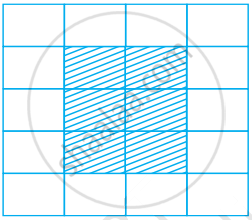Exercise | Q 76.(b) | Page 128

In a floral design made from tiles dimensions 40 cm by 60 cm (See figure), find the ratios of the area of the shaded portion to the area of the unshaded portion.Exercise | Q 77 | Page 128

In figure, what is the ratio of the areas of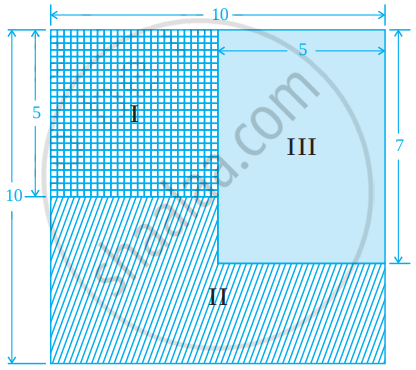(c) shaded portions I and II taken together and shaded portion III?

Exercise | Q 78 | Page 129

A car can travel 240 km in 15 litres of petrol. How much distance will it travel in 25 litres of petrol?

Exercise | Q 79.(a) | Page 129

Bachhu Manjhi earns Rs. 24000 in 8 months. At this rate, how much does he earn in one year?

Exercise | Q 79.(b) | Page 129

Bachhu Manjhi earns Rs. 24000 in 8 months. At this rate, in how many months does he earn Rs. 42000?

Exercise | Q 80 | Page 129

The yield of wheat from 8 hectares of land is 360 quintals. Find the number of hectares of land required for a yield of 540 quintals?

Exercise | Q 81 | Page 129

The earth rotates 360° about its axis in about 24 hours. By how much degree will it rotate in 2 hours?

Exercise | Q 82 | Page 129

Shivangi is suffering from anaemia as haemoglobin level in her blood is lower than the normal range. Doctor advised her to take one iron tablet two times a day. If the cost of 10 tablets is Rs. 17, then what amount will she be required to pay for her medical bill for 15 days?

Exercise | Q 83 | Page 129

The quarterly school fee in Kendriya Vidyalaya for Class VI is Rs. 540. What will be the fee for seven months?

Exercise | Q 84 | Page 129

In an election, the votes cast for two of the candidates were in the ratio 5:7. If the successful candidate received 20734 votes, how many votes did his opponent receive?

Exercise | Q 85 | Page 129

A metal pipe 3 metre long was found to weigh 7.6 kg. What would be the weight of the same kind of 7.8 m long pipe?

Exercise | Q 86 | Page 129

A recipe for raspberry jelly calls for 5 cups of raspberry juice and 2 1/2 cups of sugar. Find the amount of sugar needed for 6 cups of the juice?

Exercise | Q 87 | Page 129

A farmer planted 1890 tomato plants in a field in rows each having 63 plants. A certain type of worm destroyed 18 plants in each row. How many plants did the worm destroy in the whole field?

Exercise | Q 88 | Page 130

Length and breadth of the floor of a room are 5 m and 3 m, respectively. forty tiles, each with area 1/16 m2 are used to cover the floor partially. Find the ratio of the tiled and the non tiled portion of the floor.

Exercise | Q 89 | Page 130

A carpenter had a board which measured 3 m × 2 m. She cut out a rectangular piece of 250 cm × 90 cm. What is the ratio of the area of cut out piece and the remaining piece?

## Chapter 8: Ratio and Proportion

Exercise## NCERT solutions for Mathematics Exemplar Class 6 chapter 8 - Ratio and Proportion

NCERT solutions for Mathematics Exemplar Class 6 chapter 8 (Ratio and Proportion) include all questions with solution and detail explanation. This will clear students doubts about any question and improve application skills while preparing for board exams. The detailed, step-by-step solutions will help you understand the concepts better and clear your confusions, if any. Shaalaa.com has the CBSE Mathematics Exemplar Class 6 solutions in a manner that help students grasp basic concepts better and faster.

Further, we at Shaalaa.com provide such solutions so that students can prepare for written exams. NCERT textbook solutions can be a core help for self-study and acts as a perfect self-help guidance for students.

Concepts covered in Mathematics Exemplar Class 6 chapter 8 Ratio and Proportion are Concept of Ratio, Concept of Proportion, Concept of Unitary Method.

Using NCERT Class 6 solutions Ratio and Proportion exercise by students are an easy way to prepare for the exams, as they involve solutions arranged chapter-wise also page wise. The questions involved in NCERT Solutions are important questions that can be asked in the final exam. Maximum students of CBSE Class 6 prefer NCERT Textbook Solutions to score more in exam.

Get the free view of chapter 8 Ratio and Proportion Class 6 extra questions for Mathematics Exemplar Class 6 and can use Shaalaa.com to keep it handy for your exam preparation